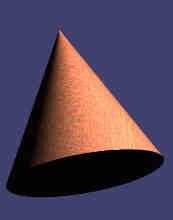# Cones - Overview

Author:
Christine
Topic:
ConeCone Facts: Notice these interesting things:
• It has a flat base
• It has one curved side• The pointy end of a cone is called the apex
• The flat part is the base
• An object shaped like a cone is said to be conical
Surface Area of a Cone:The Surface Area has two parts:
• The Base Area = π × r2
• The Side Area = π × r × s
Which together makes: Surface Area = π × r × (r + s) Note: we can calculate s = √(r2+h2) Example: h = 7 and r = 2 Surface Area of Base= π × r2 ﻿ ﻿ ﻿ ﻿  = π × 22   ﻿ ﻿ ﻿ ﻿ = 4π   ﻿ ﻿ ﻿ ﻿ ≈ 12.57   Surface Area of Side= π × r × √(r2+h2)   ﻿ ﻿ ﻿ ﻿ = π × 2 × √(22+72)   ﻿ ﻿ ﻿ ﻿ = π × 2 × √(4+49)   ﻿ ﻿ ﻿ ﻿ = 2π√(53)   ﻿ ﻿ ﻿ ﻿ ≈ 45.74 Total Surface Area ≈ 12.57 + 45.74 ≈ 58.31 Volume of a Cone: Volume = 1/3 π × r× h Example: h = 7 and r = 2 Volume= 13 π × r2 × h   ﻿ = 13 π × 22 × 7   ﻿ = 283 π   ﻿ ≈ 29.32 Volume of a Cone vs Cylinder:The volume formulas for cones and cylinders are very similar:
 The volume of a cylinder is: π × r2 × h The volume of a cone is: 1/3 π × r2 × h
So a cone's volume is exactly one third ( 13 ) of a cylinder's volume. Different Shaped Cones:Construction ConeThis is almost a cone, but the top is chopped off (called a "truncated cone"). Also it has a wider base added so it doesn't fall over!